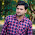# Computer Science and Information Technology

GATE Syllabus

• Discrete and Engineering mathematics.
Mathematical logic:- Propositional and first-order logic.

Set Theory and Algebra:- Sets, relations, functions, partial orders, and lattices. Groups.

Combinatorics:- counting, recurrence relations, generating functions.

Graph Theory:- Connectivity, matching, coloring.

Probability:-Random variables. Uniform, normal, exponential, Poisson and binomial distributions. Mean, median, mode and standard deviation. Conditional probability and Bayes theorem.

Linear Algebra:- Matrices, determinants, System of linear equations, eigenvalues and eigenvectors, LU decomposition.

• # General Aptitude.

Verbal Ability:- English grammar, sentence completion, verbal analogies, word groups, Instructions, critical reasoning, and verbal deduction.

Numerical Ability:- Numerical computation, numerical estimation, numerical reasoning and data Interpretation

• # Computer Science and Information Technology(core Subjects).

Theory of Computation:- Regular expressions and finite automata. Context-free grammars and push-down automata. Regular and context-free languages, pumping lemma. Turing machines and undecidability.

Digital Logic:- Boolean algebra. Combinational and sequential circuits. Minimization. Number representations and computer arithmetic (fixed and floating-point).

Computer Organization and Architecture:- Machine instructions and addressing modes. ALU, data‐path and control unit. Instruction pipelining. Memory hierarchy: cache, main memory, and secondary storage; I/O interface (Interrupt and DMA mode).

Programming and Data Structures:- Programming in C. Recursion. Arrays, stacks, queues, linked lists, trees, binary search trees, binary heaps, graphs.

Algorithms:-Searching, sorting, hashing. Asymptotic worst-case time and space complexity. Algorithm design techniques: greedy, dynamic programming and divide‐and‐conquer. Graph search, minimum spanning trees, shortest paths.

Compiler Design:-Lexical analysis, parsing, syntax-directed translation. Runtime environments. Intermediate code generation.

Operating System:- Processes, threads, inter‐process communication, concurrency, and synchronization. Deadlock. CPU scheduling. Memory management and virtual memory. File systems.

Databases:- ER‐model. Relational model: relational algebra, tuple calculus, SQL. Integrity constraints, normal forms. File organization, indexing (e.g., B and B+ trees). Transactions and concurrency control.

Computer Networks:- The concept of layering. LAN technologies (Ethernet). Flow and error control techniques, switching. IPv4/IPv6, routers and routing algorithms (distance vector, link state). TCP/UDP and sockets, congestion control. Application layer protocols (DNS, SMTP, POP, FTP, HTTP). Basics of Wi-Fi. Network security: authentication, basics of f public k, y and private key cryptography, digital signatures and certificates, firewalls.

GATE Syllabus

#### 1 comment:

1.niveeeeeeeeeeeeeeeeeeeeee post